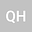loading page

Analysis of long-term solution of chemotactic model with indirect signal consumption in three-dimensional case
•## Abstract

In this paper, we consider the chemotaxis model u_t&=\Delta u-\nabla\cdot(u\nabla v),& \qquad x\in\Omega,\,t>0,v_t&=\Delta v-vw,& \qquad x\in\Omega,\,t>0,w_t&=-\delta w+u,& \qquad x\in\Omega,\,t>0,under homogeneous Neumann boundary conditions in a bounded and convex domain $\Om\subset \mathbb{R}^3$ with smooth boundary, where $\delta>0$ is a given parameter. It is shown that for arbitrarily large initial data, this problem admits at least one global weak solution for which there exists $T>0$ such that the solution $(u,v,w)$ is bounded and smooth in $\Om\times(T,\infty)$. Furthermore, it is asserted that such solutions approach spatially constant equilibria in the large time limit.

#### Peer review status:IN REVISION

14 Feb 2021Submitted to Mathematical Methods in the Applied Sciences
15 Feb 2021Assigned to Editor
15 Feb 2021Submission Checks Completed
20 Feb 2021Reviewer(s) Assigned
29 May 2021Review(s) Completed, Editorial Evaluation Pending
30 May 2021Editorial Decision: Revise Major